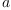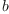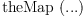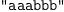login  home  contents  what's new  discussion  bug reports help  links  subscribe  changes  refresh  edit

# Edit detail for SandBox Monoid revision 4 of 4

 1 2 3 4 Editor: Bill Page Time: 2015/04/10 17:08:31 GMT+0 Note: format

added:
)abbrev category MM MyMonoid


)abbrev category MM MyMonoid
)if false
-- Does not work now
Monoid(m:Symbol,u:Symbol): Category == with()
m: (%,%) -> %       ++ returns the product of x and y
u: () -> %          ++ unit
associative(m)      ++ m(a,m(b,c)) = m(m(a,b),c)
identity(u)         ++ m(a,u) = m(u,a) = a
Group(m:Symbol,inv:Symbol,u:Symbol): Category == Monoid(m,u) with
inv: % -> %         ++ inverse
inverse(m,inv)      ++ m(inv(a),a) = m(a,inv(a)) = u
AbelianGroup(m:Symbol,inv:Symbol,u:Symbol): Category
== Group(m,inv,u) with
commutative(m)       ++ m(a,b) = m(b,a)
Ring(s:Symbol,inv:Symbol,z:Symbol, m:Symbol,u:Symbol): Category
== Join(AbelianGroup(s,inv,z),Monoid(m,u)) with
distributes(m,s)     ++ m(a,s(b,c)) = s(m(a,b),m(a,c))
++ m(s(a,b),c) = s(m(a,c),m(b,c))
)endif
   Compiling FriCAS source code from file
using old system compiler.
MM abbreviates category MyMonoid

fricas
)sh Ring(+,-,"0"::Symbol,*,"1"::Symbol)
The constructor Ring takes 0 arguments and you have given  5  .

Let's try this ...

fricas
)sh Ring(a,b,c,d,e)
The constructor Ring takes 0 arguments and you have given  5  .

Interesting! Is it somewhere written that "has" can have a category as its first argument?

OK, we are going to implement...

)abbrev domain MYINT MyInteger
Rep:=Integer
a(x: %, y: %): % == x
b(x: %): % == x
c(): % == 0 pretend %
d(x: %, y: %): % == x
e(): % == 0 pretend %
   Compiling FriCAS source code from file
using old system compiler.
MYINT abbreviates domain MyInteger
------------------------------------------------------------------------
initializing NRLIB MYINT for MyInteger
compiling into NRLIB MYINT
>> System error:
invalid number of arguments: 5

Oops, that's not so easy. Have I made some error? (You originally wrote begin{axiom} but this is spad code.

Unfortunately, this won't work. For example in the above definition of Monoid, we are effectively creating to different things with the same name, but different types. We have an m of type Symbol, and another one of type (%,%)->%. As soon as we add a default implementation, this mistake surfaces:

)abbrev category MYMON MyMonoid
MyMonoid(m:Symbol): Category == with
m:(%,%) -> %
square:% -> %
square(a:%):% == m(a,a)$% spad  Compiling FriCAS source code from file /var/lib/zope2.10/instance/axiom-wiki/var/LatexWiki/1161784151565736029-25px005.spad using old system compiler. MYMON abbreviates category MyMonoid ------------------------------------------------------------------------ initializing NRLIB MYMON for MyMonoid compiling into NRLIB MYMON ;;; *** |MyMonoid| REDEFINED Time: 0 SEC. MYMON- abbreviates domain MyMonoid& ------------------------------------------------------------------------ initializing NRLIB MYMON- for MyMonoid& compiling into NRLIB MYMON- compiling exported square : S -> S Time: 0 SEC. (time taken in buildFunctor: 0) ;;; *** |MyMonoid&| REDEFINED Time: 0 SEC. Cumulative Statistics for Constructor MyMonoid& Time: 0 seconds finalizing NRLIB MYMON- Processing MyMonoid& for Browser database: --->-->MyMonoid&(constructor): Not documented!!!! --->-->MyMonoid&((m (% % %))): Not documented!!!! --->-->MyMonoid&((square (% %))): Not documented!!!! --->-->MyMonoid&(): Missing Description ; compiling file "/var/aw/var/LatexWiki/MYMON-.NRLIB/MYMON-.lsp" (written 04 APR 2022 05:29:01 PM): ; /var/aw/var/LatexWiki/MYMON-.NRLIB/MYMON-.fasl written ; compilation finished in 0:00:00.008 ------------------------------------------------------------------------ MyMonoid& is now explicitly exposed in frame initial MyMonoid& will be automatically loaded when needed from /var/aw/var/LatexWiki/MYMON-.NRLIB/MYMON- finalizing NRLIB MYMON Processing MyMonoid for Browser database: --->-->MyMonoid(constructor): Not documented!!!! --->-->MyMonoid((m (% % %))): Not documented!!!! --->-->MyMonoid((square (% %))): Not documented!!!! --->-->MyMonoid(): Missing Description ; compiling file "/var/aw/var/LatexWiki/MYMON.NRLIB/MYMON.lsp" (written 04 APR 2022 05:29:01 PM): ; /var/aw/var/LatexWiki/MYMON.NRLIB/MYMON.fasl written ; compilation finished in 0:00:00.003 ------------------------------------------------------------------------ MyMonoid is now explicitly exposed in frame initial MyMonoid will be automatically loaded when needed from /var/aw/var/LatexWiki/MYMON.NRLIB/MYMON spad )abbrev domain WORD Word Word(c:Symbol): MyMonoid(c) with coerce:String -> % coerce:% -> OutputForm == add Rep := String coerce(a:String):% == a pretend % coerce(x:%):OutputForm == message(x pretend String)$OutputForm
c(a:%, b:%):% == concat(a::Rep, b::Rep)
   Compiling FriCAS source code from file
using old system compiler.
WORD abbreviates domain Word
------------------------------------------------------------------------
initializing NRLIB WORD for Word
compiling into NRLIB WORD
compiling exported coerce : String -> $WORD;coerce;S$;1 is replaced by a
Time: 0 SEC.
compiling exported coerce : $-> OutputForm Time: 0 SEC. compiling exported c : ($,$) ->$
WORD;c;3$;3 is replaced by STRCONC Time: 0 SEC. (time taken in buildFunctor: 0) ;;; *** |Word| REDEFINED ;;; *** |Word| REDEFINED Time: 0 SEC. Warnings:  coerce: pretend$ -- should replace by @
Cumulative Statistics for Constructor Word
Time: 0 seconds
finalizing NRLIB WORD
Processing Word for Browser database:
--->-->Word(constructor): Not documented!!!!
--->-->Word((coerce (% (String)))): Not documented!!!!
--->-->Word((coerce ((OutputForm) %))): Not documented!!!!
--->-->Word(): Missing Description
; compiling file "/var/aw/var/LatexWiki/WORD.NRLIB/WORD.lsp" (written 04 APR 2022 05:29:01 PM):
; /var/aw/var/LatexWiki/WORD.NRLIB/WORD.fasl written
; compilation finished in 0:00:00.012
------------------------------------------------------------------------
Word is now explicitly exposed in frame initial
Word will be automatically loaded when needed from
/var/aw/var/LatexWiki/WORD.NRLIB/WORD

fricas
)sh Word
Word(c: Symbol) is a domain constructor
Abbreviation for Word is WORD
This constructor is exposed in this frame.
------------------------------- Operations --------------------------------
c : (%, %) -> %                       coerce : % -> OutputForm
coerce : String -> %                  square : % -> %

fricas
)sh Word("p"::Symbol)
Word(p) is a domain constructor.
Abbreviation for Word is WORD
This constructor is exposed in this frame.
3 Names for 4 Operations in this Domain.
------------------------------- Operations --------------------------------
coerce : % -> OutputForm              coerce : String -> %
p : (%, %) -> %                       square : % -> %
w1:="a"::Word("p"::Symbol)(1)
Type: Word(p)
fricas
w2:="b"::Word("p"::Symbol)(2)
Type: Word(p)
fricas
p(w1,w2)$Word("p"::Symbol) The function p is not implemented in Word(p) . What we really would like to have would be something along the lines of: spad )abbrev category MYMON1 MyMonoid1 MyMonoid1(S:SetCategory, m: (S,S)-> S): Category == with m: (S,S)-> S square: S -> S add m square a == m(a,a) spad  Compiling FriCAS source code from file /var/lib/zope2.10/instance/axiom-wiki/var/LatexWiki/6825787861264248532-25px008.spad using old system compiler. MYMON1 abbreviates category MyMonoid1 ------------------------------------------------------------------------ initializing NRLIB MYMON1 for MyMonoid1 compiling into NRLIB MYMON1 ;;; *** |MyMonoid1| REDEFINED Time: 0 SEC. MYMON1- abbreviates domain MyMonoid1& ------------------------------------------------------------------------ initializing NRLIB MYMON1- for MyMonoid1& compiling into NRLIB MYMON1- >> System error: The value |m| is not of type LIST. fricas )sh MyMonoid1 MyMonoid1 is a category constructor Abbreviation for MyMonoid1 is MYMON1 This constructor is not exposed in this frame. ------------------------------- Operations -------------------------------- fricas )set functions compile on m1:=(a:String,b:String):String+->concat(a,b)(3) Type: ((String, String) -> String) fricas m1("aaa","bbb")(4) Type: String fricas )sh MyMonoid1(String,m1) MyMonoid1 is an unknown constructor and so is unavailable. Did you mean to use -> but type something different instead? Then:  Word: MyMonoid1(String,(a:String,b:String):String+->concat(a,b)) with coerce: String -> % == add Rep := String coerce(a: String): % == a c(a:%, b:%):% == concat(a::Rep, b::Rep)  However, there are two problems here: • it is not possible to refer to the category to be defined in the parameter, as in MyMonoid(m: (%,%)-> %) • it is not possible to refer to an operation which is not yet defined as in Word: MyMonoid(c) with Martin scope of names in default implementation of categories --Bill Page, Thu, 02 Mar 2006 09:42:18 -0600 reply Martin wrote: Unfortunately, this won't work. For example in the above definition of Monoid, we are effectively creating to different things with the same name, but different types. We have an m of type Symbol, and another one of type (%,%)->%. I agree that there is a scope issue here. Perhaps it comes from the idea of allowing default implementation as part of the category definition. But I think the proper semantics are quite easy to define. The exports need to have priority over the parameters. The m of type Symbol is not exported by MyMonoid but the m of type (%,%)-> % is exported. The m in the implementation m(a,a) should refer to m that is being exported. So I think this is a compiler error. generic monoids in Aldor --Bill Page, Fri, 03 Mar 2006 15:46:42 -0600 reply Based on an idea posted to this page by Martin Rubey, I have constructed what I think is a good facsimile of a generic monoid. I think think that this construction is only possible in Aldor. The idea is basically to specify a monoid as a tuple in the usual manner, consisting of a set S, an associative binary operator m and unit u. In this example MyMonoid? exports two operations and a constant: * for the binary operation in the monoid (whatever it happens to be), ^ for repeated * repeated n times, and 1 denoting the ring identity. This is rather different that the notion that we started with in this thread - in fact more or less the opposite: from any more complex domain we can extract the monoid part. Of course this is fundamentally very simple, just as it should be. :) aldor #include "axiom" define associative(S:SetCategory, m:(S,S)->S):Category == with { default ForAll(a:S,b:S,c:S):Boolean == m(a,m(b,c)) = m(m(a,b),c); }; define identity(S:SetCategory, m:(S,S)->S, u:S): Category == with { default ForAll(a:S):Boolean == m(a,u) = a and m(u,a) = a; }; MyMonoid(S:SetCategory, m:(S,S)->S, u:S): with { associative(S,m); identity(S,m,u); *:(%,%) -> %; 1: %; ^:(%,NonNegativeInteger) -> %; ++ a^0 = 1, a*a^n = a^(n+1) coerce: S -> %; coerce: % -> OutputForm; } == add { Rep == S; coerce(a: S): % == per(a); coerce(x:%):OutputForm == coerce(rep(x))$S;
-- product
(a:%) * (b:%):% == per(m(rep(a),rep(b)));
-- Repeated squaring
(x:%)^(n:NonNegativeInteger):% == {
import from Integer,NonNegativeInteger;
(n = 0) => return 1;
odd?(n::Integer) => return x*(x*x)^shift(n,-1);
return (x*x)^shift(n,-1);
};
-- unit
1 == per(u);
}
aldor
   Compiling FriCAS source code from file
/var/lib/zope2.10/instance/axiom-wiki/var/LatexWiki/mymonoid.as
using Aldor compiler and options
-O -Fasy -Fao -Flsp -lfricas -Mno-ALDOR_W_WillObsolete -DFriCAS -Y $FRICAS/algebra -I$FRICAS/algebra
Use the system command )set compiler args to change these
options.
The )library system command was not called after compilation.

This is how the MyMonoid domain looks to the Axiom interpreter.

fricas
)sh MyMonoid
MyMonoid(m: Symbol) is a category constructor
Abbreviation for MyMonoid is MYMON
This constructor is exposed in this frame.
------------------------------- Operations --------------------------------
m : (%, %) -> %                       square : % -> %

fricas
)sh MyMonoid(String,concat,"")
The constructor MyMonoid takes 1 argument and you have given  3  .

Here is Martin Rubey's String monoid example:

fricas
w1:="a"::MyMonoid(String,concat,"")
The constructor MyMonoid takes 1 argument and you have given  3  .

And of course we can construct a large number of other examples.

fricas
i1:=[1,2]::MyMonoid(List INT, concat,[])
The constructor MyMonoid takes 1 argument and you have given  3  .

fricas
i1:=10::MyMonoid(INT, +, 0)
The constructor MyMonoid takes 1 argument and you have given  3  .

fricas
i1:=10.1::MyMonoid(Float, *, 1)
The constructor MyMonoid takes 1 argument and you have given  3  .

Now try to define a group.

aldor
#include "axiom"
#library MyMonoid "mymonoid.ao";
import from MyMonoid;
define inverse(S:SetCategory, m:(S,S)->S, inv:S->S, u:S): Category == with {
default ForAll(a:S):Boolean == m(inv(a),a)=u and m(a,inv(a))=u;
};
MyGroup(S:SetCategory, m:(S,S)->S, inv:S->S, u:S): with {
associative(S,m);
identity(S,m,u);
inverse(S,m,inv,u);
~:% -> %;
*:(%,%) -> %;
1: %;
^:(%,NonNegativeInteger) -> %;  ++ a^0 = 1, a*a^n = a^(n+1)
coerce: S -> %;
coerce: % -> OutputForm;
Rep == S;
coerce(a: S): % == per(a);
coerce(x:%):OutputForm == coerce(rep(x))$S; -- inverse ~(a:%):% == per(inv(rep(a))); } aldor  Compiling FriCAS source code from file /var/lib/zope2.10/instance/axiom-wiki/var/LatexWiki/mygroup.as using Aldor compiler and options -O -Fasy -Fao -Flsp -lfricas -Mno-ALDOR_W_WillObsolete -DFriCAS -Y$FRICAS/algebra -I $FRICAS/algebra Use the system command )set compiler args to change these options. The )library system command was not called after compilation. This is how the MyGroup domain looks to the Axiom interpreter. fricas )sh MyGroup The )show system command is used to display information about types or partial types. For example, )show Integer will show information about Integer . MyGroup is not the name of a known type constructor. If you want to see information about any operations named MyGroup , issue )display operations MyGroup  fricas )sh MyGroup(INT,+,-,0) The )show system command is used to display information about types or partial types. For example, )show Integer will show information about Integer . MyGroup is not the name of a known type constructor. If you want to see information about any operations named MyGroup , issue )display operations MyGroup INT is not the name of a known type constructor. If you want to see information about any operations named INT , issue )display operations INT + is not the name of a known type constructor. If you want to see information about any operations named + , issue )display operations + - is not the name of a known type constructor. If you want to see information about any operations named - , issue )display operations - 0 is not the name of a known type constructor. If you want to see information about any operations named 0 , issue )display operations 0 For example: fricas x:=3::MyGroup(INT,+,-,0) There are no library operations named MyGroup Use HyperDoc Browse or issue )what op MyGroup to learn if there is any operation containing " MyGroup " in its name. Cannot find a definition or applicable library operation named MyGroup with argument type(s) Type Variable(+) Variable(-) NonNegativeInteger Perhaps you should use "@" to indicate the required return type, or "$" to specify which version of the function you need.

A different issue? --wyscc, Wed, 08 Mar 2006 12:35:43 -0600 reply
I am not impressed. However, this kind of finessed the whole issue of notation and neglected the dual inheritance situation, and I expect one will get into trouble when one wants to go on to MyRing. Any domain in MyRing should work without unnecessary package calls in the Interpreter. All this mechanism did was passing a function m to constructors, here to be used as the monoid categorically defined multiplication. This technique has been used before, in Axiom, in GDMP. The notation in an Interpreter session is still *, the operator declared in MyMonoid. So even though we have MyMonoid(INT,+,0) and we can compute using i1*i2 to really get the sum of i1 and i2, this is not using + notation. Notice also that MyGroup should have been implemented as a category constructor. In )sh MyGroup, the group operation is *, the unit is 1 and the inverse is - (which is incompatible with * and wrong, since a group should not be commutative in general). But these last two comments are minor quibbles and easily corrected.

I indicated before that I believe one needs to make some deeper changes to implement inheritance that would support notational changes in a way such that multiple inheritance of MyMonoid to the same domain constructor can distinguish the operators. Any proposed solution under the currently available systems, if possible at all, should include MyRing (as a category, not domain) and MyInteger as a domain in MyRing.

I am more interested in the associative category and the ForAll in Aldor. How exactly does that differ from Axiom? ForAll seems to be only a case by case verification, given actual elements of the domain.

William

Re: A different issue? --Bill Page, Wed, 08 Mar 2006 21:45:13 -0600 reply
William, it seems to me that you are easily not impressed ;) But thanks for your comments.

I agree that the second half of this page implements a different structure than the first half - I said as much above. It is in a formal sense the exact opposite thing. But opposites can be useful. I am working out the details here because I am hoping that in the end we can see the issue of inheritance more precisely as the dual to this construction.

The point here is that MyGroup(INT,+,-,0) declares that this combination of domain, operations and constant constitutes a group. It does not attempt to construct this group in INT but rather it extracts this part of INT as a subdomain having the structure of a group:

    MyGroup(INT,+,-,0) >-----> INT
with *, ~, 1               with +, -, 0, *, /, 1,  ...


In categorical terms both MyMonoid and MyGroup are monomorhic functors, i.e. subdomain constructors.

(Note that I changed the notation for inverse in MyGroup to ~ so that perhaps it is less confusing.)

MyGroup is not implemented as a category constructor because as I said, the intent here is not to construct MyInteger by inheritance. In this case the implementation of Integer is given and we are simply identifying parts of it.

Inheritance does require something similiar:

    MyInteger: Join(Group(%,+,-,0), Monoid(%, *, 1), ...
with *, ~, 1    with *, 1


In this case the categories Group, Monoid, etc. are given and we wish to provide new names for their operations in MyInteger as suggested by Ralf. Unfortunately as Martin demonstrated on the first part of this page, the SPAD compiler does not compile it correctly. Maybe we can still find a way to do this with Aldor. I haven't given up yet, I am just working on a different aspect of the problem.

The associative category as I implemented it above in Aldor can also be written this way in SPAD. But as far as I know Aldor does not implement Axiom's axioms (assertions) so in Aldor these must be coded as categories with a possibly empty with { } clause.

ForAll is not an Aldor primitive construct, it is just a simple export that is intended to express the axioms in a manner that could be used in some kind of theorem proving subsystem (which does not exist yet :). You are right however that this could very easily be used to implement an automatic verification system. Such as system could least provide useful counter examples when the axioms fail.

I am still thinking about how best to encode these sort of axioms, so the ForAll construct above is likely to change a little.

Stay tuned to this channel ...

Define an Abelian (commutative) group:

aldor
#include "axiom"
#library MyMonoid "mymonoid.ao";
import from MyMonoid;
#library MyGroup "mygroup.ao";
import from MyGroup;
define commutative(S:SetCategory, m:(S,S)->S): Category == with {
default ForAll(a:S,b:S):Boolean == m(a,b) = m(b,a);
};
MyAbelianMonoid(S:SetCategory, s:(S,S)->S, z:S): with {
commutative(S,s);
associative(S,s);
identity(S,s,z);
+:(%,%) -> %;
0: %;
^:(%,NonNegativeInteger) -> %;  ++ a^0 = 1, a*a^n = a^(n+1)
coerce: S -> %;
coerce: % -> OutputForm;
Rep == S;
coerce(a: S): % == per(a);
coerce(x:%):OutputForm == coerce(rep(x))$S; -- product (a:%) + (b:%):% == per(s(rep(a),rep(b))); -- Repeated squaring (x:%)^(n:NonNegativeInteger):% == { import from Integer,NonNegativeInteger; (n = 0) => return 0; odd?(n::Integer) => return x+(x+x)^shift(n,-1); return (x+x)^shift(n,-1); }; -- unit 0 == per(z); } MyAbelianGroup(S:SetCategory, s:(S,S)->S, inv:S->S, z:S): with { commutative(S,s); associative(S,s); identity(S,s,z); inverse(S,s,inv,z); -:% -> %; +:(%,%) -> %; 0: %; ^:(%,NonNegativeInteger) -> %; ++ a^0 = 1, a*a^n = a^(n+1) coerce: S -> %; coerce: % -> OutputForm; } == MyAbelianMonoid(S,s,z) add { Rep == S; coerce(a: S): % == per(a); coerce(x:%):OutputForm == coerce(rep(x))$S;
-- inverse
-(a:%):% == per(inv(rep(a)));
}
aldor
   Compiling FriCAS source code from file
/var/lib/zope2.10/instance/axiom-wiki/var/LatexWiki/myabeliangroup.as
using Aldor compiler and options
-O -Fasy -Fao -Flsp -lfricas -Mno-ALDOR_W_WillObsolete -DFriCAS -Y $FRICAS/algebra -I$FRICAS/algebra
Use the system command )set compiler args to change these
options.
The )library system command was not called after compilation.

MyAbelianMonoid? is a MyMonoid?. MyAbelianGroup? is a MyGroup? and a MyMonoid?.

fricas
)sh MyAbelianMonoid
The )show system command is used to display information about types
or partial types. For example, )show Integer will show
MyAbelianMonoid is not the name of a known type constructor. If
you want to see information about any operations named
MyAbelianMonoid , issue
)display operations MyAbelianMonoid 
fricas
)sh MyMonoid(MyAbelianMonoid(INT,+,0),+,0)
The constructor MyMonoid takes 1 argument and you have given  3  .

Define a ring:

aldor
#include "axiom"
#library MyMonoid "mymonoid.ao";
import from MyMonoid;
#library MyGroup "mygroup.ao";
import from MyGroup;
#library MyAbelianGroup "myabeliangroup.ao";
import from MyAbelianGroup;
define distributes(S:SetCategory, m:(S,S)->S, s:(S,S)->S): Category == with {
default ForAll(a:S,b:S,c:S):Boolean ==
m(a,s(b,c)) = s(m(a,b),m(a,c)) and
m(s(a,b),c) = s(m(a,c),m(b,c));
};
MyRing(S:SetCategory, s:(S,S)->S, inv:S->S, z:S, m:(S,S)->S, u:S): with {
distributes(S,m,s);
associative(S,s);
commutative(S,s);
identity(S,s,z);
inverse(S,m,inv,z);
associative(S,m);
identity(S,m,u);
*:(%,%) -> %;
1: %;
+:(%,%) -> %;
0: %;
^:(%,NonNegativeInteger) -> %;  ++ a^0 = 1, a*a^n = a^(n+1)
coerce: S -> %;
coerce: % -> OutputForm;
} == {
MyAbelianGroup(S,s,inv,z);
MyMonoid(S,m,u);
} add { }
aldor
   Compiling FriCAS source code from file
/var/lib/zope2.10/instance/axiom-wiki/var/LatexWiki/4246596118391608395-25px022.as
using Aldor compiler and options
-O -Fasy -Fao -Flsp -lfricas -Mno-ALDOR_W_WillObsolete -DFriCAS -Y $FRICAS/algebra -I$FRICAS/algebra
Use the system command )set compiler args to change these
options.
The )library system command was not called after compilation.

It looks like this.

fricas
)sh MyRing
The )show system command is used to display information about types
or partial types. For example, )show Integer will show
MyRing is not the name of a known type constructor. If you want
to see information about any operations named MyRing , issue
)display operations MyRing

Integer is a MyRing?.

fricas
x3:=3::MyRing(Integer,+,-,0,*,1)
There are no library operations named MyRing
Use HyperDoc Browse or issue
)what op MyRing
to learn if there is any operation containing " MyRing " in its
name.
Cannot find a definition or applicable library operation named
MyRing with argument type(s)
Type
Variable(+)
Variable(-)
NonNegativeInteger
Variable(*)
PositiveInteger
Perhaps you should use "@" to indicate the required return type,
or "\$" to specify which version of the function you need.

Martin Rubey discovered a way to use the Aldor extend construct to add Monoid(Integer,*,1) as a category to an existing domain, thus in principle also allowing this to be distinguished from Monoid(Integer,+,0) in a if ... has ... statement. But there may be problems. See: SandBox Monoid Extend.

But an AbelianDuck? really is a Duck! --Bill Page, Sun, 27 Jul 2008 13:40:55 -0700 reply
SandBoxAbelianDuck